# On the refinements of the Jensen-Steffensen inequality

## Abstract

In this paper, we extend some old and give some new refinements of the Jensen-Steffensen inequality. Further, we investigate the log-convexity and the exponential convexity of functionals defined via these inequalities and prove monotonicity property of the generalized Cauchy means obtained via these functionals. Finally, we give several examples of the families of functions for which the results can be applied.

2010 Mathematics Subject Classification. 26D15.

## 1. Introduction

One of the most important inequalities in mathematics and statistics is the Jensen inequality (see [, p.43]).

Theorem 1.1. Let I be an interval in and f : I be a convex function. Let n ≥ 2, x = (x1, ..., x n ) In and p = (p1, ..., p n ) be a positive n-tuple, that is, such that p i > 0 for i = 1, ..., n. Then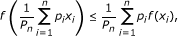(1)

Where(2)

If f is strictly convex, then inequality (1) is strict unless x1 = = x n .

The condition "p is a positive n-tuple" can be replaced by "p is a non-negative n-tuple and P n > 0". Note that the Jensen inequality (1) can be used as an alternative definition of convexity.

It is reasonable to ask whether the condition "p is a non-negative n-tuple" can be relaxed at the expense of restricting x more severely. An answer to this question was given by Steffensen  (see also [, p.57]).

Theorem 1.2. Let I be an interval in and f : I be a convex function. If x = (x1, ..., x n ) In is a monotonic n-tuple and p = (p1, ..., p n ) a real n-tuple such that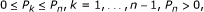(3)

is satisfied, where P k are as in (2), then (1) holds. If f is strictly convex, then inequality (1) is strict unless x1 = = x n .

Inequality (1) under conditions from Theorem 1.2 is called the Jensen-Steffensen inequality. A refinement of the Jensen-Steffensen inequality was given in  (see also [, p.89]).

Theorem 1.3. Let x and p be two real n-tuples such that ax1x n b and (3) hold. Then for every convex function f : [a, b] →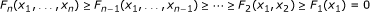(4)

holds, where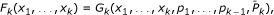(5)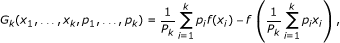(6)

P k are as in (2) and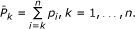(7)

Note that the function G n defined in (6) is in fact the difference of the right-hand and the left-hand side of the Jensen inequality (1).

In this paper, we present a new refinement of the Jensen-Steffensen inequality, related to Theorem 1.3. Further, we investigate the log-convexity and the exponential convexity of functionals defined as differences of the left-hand and the right-hand sides of these inequalities. We also prove monotonicity property of the generalized Cauchy means obtained via these functionals. Finally, we give several examples of the families of functions for which the obtained results can be applied.

In what follows, I is an interval in , P k are as in (2) and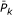are as in (7). Note that if (3) is valid, since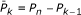, it follows thatsatisfy (3) as well.

## 2. New refinement of the Jensen-Steffensen inequality

The aim of this section is to give a new refinement of the Jensen-Steffensen inequality. In the proof of this refinement, the following result is needed (see [, p.2]).

Proposition 2.1. If f is a convex function on an interval I and if x1y1, x2y2, x1x2, y1y2, then the following inequality is valid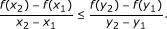(8)

If the function f is concave, the inequality reverses.

The main result states.

Theorem 2.2. Let x = (x1, ..., x n ) In be a monotonic n-tuple and p = (p1, ..., p n ) a real n-tuple such that (3) holds. Then for a convex function f : I we have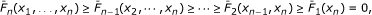(9)

where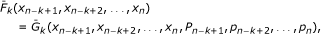(10)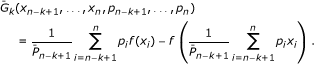(11)

For a concave function f, the inequality signs in (9) reverse.

Proof. The claim is that for a convex function f,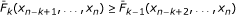holds for every k = 2, ..., n. This inequality is equivalent to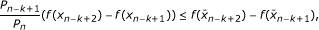(12)

where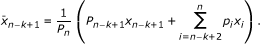If x is increasing then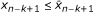, while if x is decreasing thenfor every k. Furthermore, without loss of generality, we can assume that x is strictly monotonic and that 0 < P k < P n for k = 1, ..., n - 1. Now, applying (8) for a convex function f when x is strictly increasing yields inequality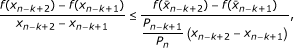while if x is strictly decreasing we get inequality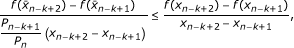both of which are equivalent to (12). If f is concave, the inequalities reverse. Thus, the proof is complete.   □

Remark 2.3. A slight extension of the proof of Theorem 1.3 inshows that Theorem 1.3 remains valid if the n-tuple x is assumed to be monotonic instead of increasing. The proof is in fact analogous to the proof of Theorem 2.2.

Let us observe inequalities (4) and (9). Motivated by them, we define two functionals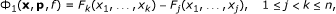(13)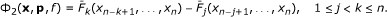(14)

where functions F k and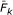are as in (5) and (10), respectively, x = (x1, ..., x n ) In is a monotonic n-tuple and p = (p1, ..., p n ) is a real n-tuple such that (3) holds. If function f is convex on I, then Theorems 1.3 and 2.2, joint with Remark 2.3, imply that Φ i (x, p, f) ≥ 0, i = 1, 2.

Now, we give mean value theorems for the functionals Φ i , i = 1, 2.

Theorem 2.4. Let x = (x1, ..., x n ) [a, b] n be a monotonic n-tuple and p = (p1, ..., p n ) a real n-tuple such that (3) holds. Let f C2[a, b] and Φ1and Φ2be linear functionals defined as in (13) and (14). Then there exists ξ [a, b] such that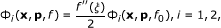(15)

where f0(x) = x2.

Proof. Analogous to the proof of Theorem 2.3 in .   □

Theorem 2.5. Let x = (x1, ..., x n ) [a, b] n be a monotonic n-tuple and p = (p1, ..., p n ) a real n-tuple such that (3) holds. Let f, g C2[a, b] be such that g"(x) ≠ 0 for every x [a, b] and let Φ1and Φ2be linear functionals defined as in (13) and (14). If Φ1and Φ2are positive, then there exists ξ [a, b] such that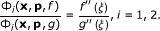(16)

Proof. Analogous to the proof of Theorem 2.4 in .   □

Remark 2.6. If the inverse of the function f"/g" exists, then (16) gives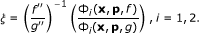(17)

## 3. Log-convexity and exponential convexity of the Jensen-Steffensen differences

We begin this section by recollecting definitions of properties which are going to be explored here and also some useful characterizations of these properties (see [, p.373]). Again, I is an open interval in .

Definition 1. A function h : I is exponentially convex on I if it is continuous and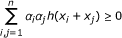holds for every n , α i and x i such that x i + x j I, i, j = 1, ..., n.

Proposition 3.1. Function h : I is exponentially convex if and only if h is continuous and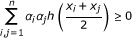holds for every n , α i and x i I, i = 1, ..., n.

Corollary 3.2. If h is exponentially convex, then the matrix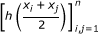is a positive semi-definite matrix. Particularly,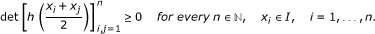Corollary 3.3. If h : I → (0, ∞) is an exponentially convex function, then h is a log-convex function, that is, for every x, y I and every λ [0, 1] we have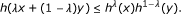Lemma 3.4. A function h : I → (0, ∞) is log-convex in the J-sense on I, that is, for every x, y I,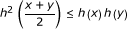holds if and only if the relation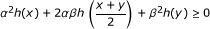holds for every α, β and x, y I.

Definition 2. The second order divided difference of a function f : [a, b] → at mutually different points y0, y1, y2 [a, b] is defined recursively by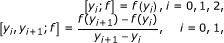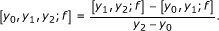(18)

Remark 3.5. The value [y0, y1, y2; f] is independent of the order of the points y0, y1and y2. This definition may be extended to include the case in which some or all the points coincide (see [, p.16]). Namely, taking the limit y1y0in (18), we get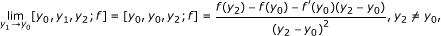provided that f' exists, and furthermore, taking the limits y i y0, i = 1, 2, in (18), we get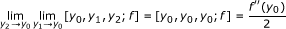provided that fexists.

Next, we study the log-convexity and the exponential convexity of functionals Φ i (i = 1, 2) defined in (13) and (14).

Theorem 3.6. Let ϒ = {f s : s I} be a family of functions defined on [a, b] such that the function s [y0, y1, y2; f s ] is log-convex in J-sense on I for every three mutually different points y0, y1, y2 [a, b]. Let Φ i (i = 1, 2) be linear functionals defined as in (13) and (14). Further, assume Φ i (x, p, f s ) > 0 (i = 1, 2) for f s ϒ. Then the following statements hold.

1. (i)

The function s Φ i (x, p, f s ) is log-convex in J-sense on I.

2. (ii)

If the function s Φ i (x, p, f s ) is continuous on I, then it is log-convex on I.

3. (iii)

If the function s Φ i (x, p, f s ) is differentiable on I, then for every s, q, u, v I such that su and qv, we have(19)

where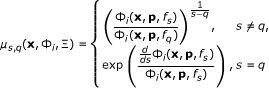(20)

and Ξ is the family functions f s belong to.

Proof. (i) For α, β and s, q I, we define a function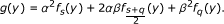Applying Lemma 3.4 for the function s [y0, y1, y2; f s ] which is log-convex in J-sense on I by assumption, yields that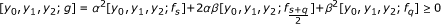which in turn implies that g is a convex function on I and therefore we have Φ i (x, p, g) ≥ 0 (i = 1, 2). Hence,Now using Lemma 3.4 again, we conclude that the function s Φ i (x, p, f s ) is log-convex in J-sense on I.

1. (ii)

If the function s Φ i (x, p, f s ) is in addition continuous, from (i) it follows that it is then log-convex on I.

2. (iii)

Since by (ii) the function s Φ i (x, p, f s ) is log-convex on I, that is, the function s log Φ i (x, p, f s ) is convex on I, applying (8) we get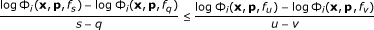(21)

for su, qv, sq, uv, and therefore conclude that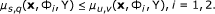If s = q, we consider the limit when qs in (21) and conclude that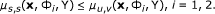The case u = v can be treated similarly.   □

Theorem 3.7. Let Ω = {f s : s I} be a family of functions defined on [a, b] such that the function s [y0, y1, y2; f s ] is exponentially convex on I for every three mutually different points y0, y1, y2 [a, b]. Let Φ i (i = 1, 2) be linear functionals defined as in (13) and (14). Then the following statements hold.

1. (i)

If n and s 1, ..., s n I are arbitrary, then the matrix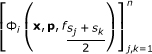is a positive semi-definite matrix for i = 1, 2. Particularly,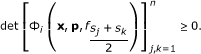(22)
1. (ii)

If the function s Φ i (x, p, f s ) is continuous on I, then it is also exponentially convex function on I.

2. (iii)

If the function s Φ i (x, p, f s ) is positive and differentiable on I, then for every s, q, u, v I such that su and qv, we have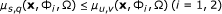(23)

where μ s, q (x, Φ i , Ω) is defined in (20).

Proof. (i) Let α j (j = 1, ..., n) and consider the function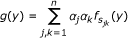for n , where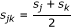, s j I, 1 ≤ j, kn and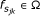. Thenand since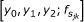is exponentially convex by assumption it follows that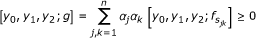and so we conclude that g is a convex function. Now we have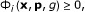which is equivalent to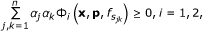which in turn shows that the matrix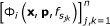is positive semi-definite, so (22) is immediate.

1. (ii)

If the function s Φ i (x, p, f s ) is continuous on I, then from (i) and Proposition 3.1 it follows that it is exponentially convex on I.

2. (iii)

If the function s Φ i (x, p, f s ) is differentiable on I, then from (ii) it follows that it is exponentially convex on I. If this function is in addition positive, then Corollary 3.3 implies that it is log-convex, so the statement follows from Theorem 3.6 (iii).   □

Remark 3.8. Note that the results from Theorem 3.6 still hold when two of the points y0, y1, y2 [a, b] coincide, say y1 = y0, for a family of differentiable functions f s such that the function s [y0, y1, y2; f s ] is log-convex in J-sense on I, and furthermore, they still hold when all three points coincide for a family of twice differentiable functions with the same property. The proofs are obtained by recalling Remark 3.5 and taking the appropriate limits. The same is valid for the results from Theorem 3.7.

Remark 3.9. Related results for the original Jensen-Steffensen inequality regarding exponential convexity, which are a special case of Theorem 3.7, were given in.

## 4. Examples

In this section, we present several families of functions which fulfil the conditions of Theorem 3.7 (and Remark 3.8) and so the results of this theorem can be applied for them.

Example 4.1. Consider a family of functions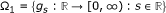defined by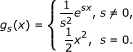We havewhich shows that g s is convex on for every s and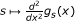is exponentially convex by Example 1 given in Jakšetić and Pečarić (submitted). From Jakšetić and Pečarić (submitted), we then also have that s [y0, y1, y2; g s ] is exponentially convex.

For this family of functions, μ s, q (x, Φ i , Ξ) (i = 1, 2) from (20) become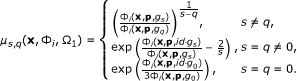Example 4.2. Consider a family of functions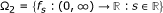defined by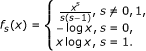Here,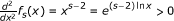which shows that f s is convex for x > 0 and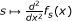is exponentially convex by Example 1 given in Jakšetić and Pečarić (submitted). From Jakšetić and Pečarić (submitted), we have that s [y0, y1, y2; f s ] is exponentially convex.

In this case, μ s, q (x, Φ i , Ξ) (i = 1, 2) defined in (20) for x j > 0 (j = 1, ..., n) areIf Φ i is positive, then Theorem 2.5 applied for f = f s Ω2and g = f q Ω2yields that there exists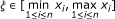such that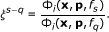Since the function ξ ξs-q is invertible for sq, we then have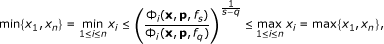(24)

which together with the fact that μ s, q (x, Φ i , Ω2) is continuous, symmetric and monotonous (by (23)), shows that μ s, q (x, Φ i , Ω2) is a mean.

Now, by substitutions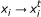,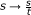,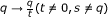from (24) we get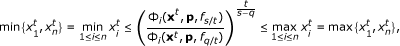where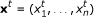.

We define a new mean (for i = 1, 2) as follows: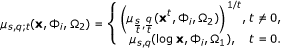(25)

These new means are also monotonous. More precisely, for s, q, u, v such that su, qv, su, qv, we have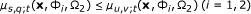(26)

We know that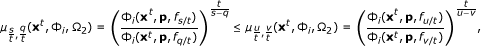for s, q, u, v I such that s/tu/t, q/tv/t and t ≠ 0, since μ s, q (x, Φ i , Ω2) are monotonous in both parameters, so the claim follows. For t = 0, we obtain the required result by taking the limit t → 0.

Example 4.3. Consider a family of functionsdefined by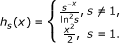Exponential convexity of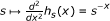on (0,∞) is given by Example 2 in Jakšetić and Pečarić (submitted).

μ s, q (x, Φ i , Ξ) (i = 1, 2) defined in (20) in this case for x j > 0 (j = 1, ..., n) are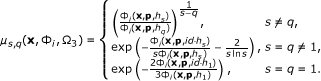Example 4.4. Consider a family of functions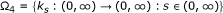defined byExponential convexity of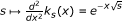on (0, ∞) is given by Example 3 in Jakšetić and Pečarić (submitted).

In this case, μ s, q (x, Φ i , Ξ) (i = 1, 2) defined in (20) for x j > 0 (j = 1, ..., n) are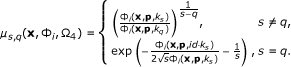## References

1. Pečarić JE, Proschan F, Tong YL: Convex Functions, Partial Orderings, and Statistical Applications. Academic Press Inc; 1992.

2. Steffensen JF: On certain inequalities and methods of approximation. J Inst Actuaries 1919, 51: 274–297.

3. Vasić PM, Pečarić JE: Sur une inegalite de Jensen-Steffensen. General Inequalities, Birkhauser Verlag, Basel 1984, 4: 87–92.

4. Khan KA, Pečarić J, Perić I: Differences of weighted mixed symmetric means and related results. J Inequal Appl 2010, 16: 289730.

5. Mitrinović DS, Pečarić J, Fink AM: Classical and New Inequalities in Analysis. Kluwer, Dordrecht; 1993.

6. Anwar M, Jakšetić J, Pečarić J, Rehman A: Exponential convexity, positive semi-definite matrices and fundamental inequalities. J Math Inequal 2010,4(2):171–189.

## Acknowledgements

This research work was partially funded by Higher Education Commission, Pakistan. The research of the first and the third author was supported by the Croatian Ministry of Science, Education and Sports, under the Research Grants 058-1170889-1050 (Iva Franjić) and 117-1170889-0888 (Josip Pečarić).

## Author information

Authors

### Competing interests

The authors declare that they have no competing interests.

### Authors' contributions

JP made the main contribution in conceiving the presented research. IF and JP worked on the results from Section 2, while SK and JP worked jointly on the results of Sections 3 and 4. IF and SK drafted the manuscript. All authors read and approved the final manuscript.

## Rights and permissions

Reprints and Permissions

Franjić, I., Khalid, S. & Pečarić, J. On the refinements of the Jensen-Steffensen inequality. J Inequal Appl 2011, 12 (2011). https://doi.org/10.1186/1029-242X-2011-12

• Accepted:

• Published:

• DOI: https://doi.org/10.1186/1029-242X-2011-12

### Keywords

• Jensen-Steffensen inequality
• refinements
• exponential and logarithmic convexity
• mean value theorems